Next: Streams Up: Representations Previous: Representations

# Terminology and Notation

The following terminology and notation is used here.

A line above a number is used to denote its negation. For example the digit minus one (-1) is usually written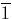.

An arrow above a digit is used to denote an infinite stream of that digit. For example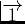denotes an infinite stream of ones.

A double colon (::) is used to separate elements of a list or stream. The last element printed represents the tail of the list or stream unless stated otherwise. Hence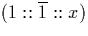is a stream whose first two elements are the digits one and minus one, and the remainder of which is a stream which would be referred to as x.

The term numeral is used to refer to the symbol or group of symbols representing a number. A number is a mathematical object, whereas a numeral is a syntactic object. When working with numerals and numbers in equations where the potential for confusion exists, we use semantic brackets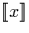to denote the number or interval represented by the numeral x.

If x is one of the representations of reals defined in this chapter,is simply the real number it represents. When working with exact reals as infinite streams, however, we often know only a finite portion of the stream. We define the range of a finite portion of a stream representing a real number to be the set of all possible reals that may be represented by a stream starting with the elements known already, but with all possible suffixes.

The symbol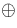is used to represent the average operation,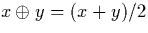. Average is a mathematical operation, but the symbol is also used to denote the result of applying an average algorithm to two numerals. In the latter case, the actual algorithm required will depend on whether the numerals are dyadic digits (see section A.2), a digit and a stream (section 3.6.2), or two streams (sections 4.2.1 and 4.3.1).

Multiplication will be represented using the symbol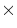, or as a single dot (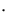). In general we will usewhen both operands are numerals in stream representation, and the dot notation for the multiplication of two numbers, or when the operands are numerals and one or both are digits.Next: Streams Up: Representations Previous: Representations
Martin Escardo
5/11/2000